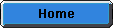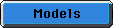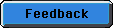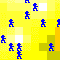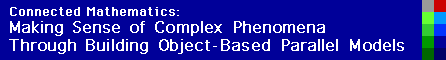Diffusion - Heat Flow

The Heat Flow models allow us to conceptualize heat flow on a thin plate where one is able to describe the exact temperatures on each side and then chart the process of the temperature distribution in real time. There are currently three Heat Flow models. Laplace is the simplest; it fixes the temperature of each edge of the plate and finds the temperature on each part of the plate when it reaches equilibrium. The Poisson model is similar but adds a heat source or sink to any point on the plate. The Diffusion model is like Laplace except that it is also "time-accurate"; that is, it simulates the actual process of gradual heat transfer through the plate over time.

Download the StarLogoT source code for the Diffusion - Heat Flow model: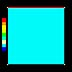In this model the internal temperature of the plate was 0, the top of the plate was 99 and the left, right, bottom sides and of the plate was 0. The red lines at the end of the model represent the path of heat flow. (292 k)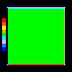In this model the internal temperature of the plate was 50, the top of the plate was 33, the left was 0, right was 66, and bottom was 99. The red lines at the end of the model represent the path of heat flow. (226 k)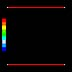In this model the internal temperature of the plate was 0, the top of the plate was 99, the left was 0, right was 0, and bottom was 99. The red lines at the end of the model represent the path of heat flow. (410 k)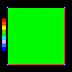In this model the internal temperature of the plate was 50, the top of the plate was 0, the left was 0, right was 99, and bottom was 99. The red lines at the end of the model represent the path of heat flow. (230 k)